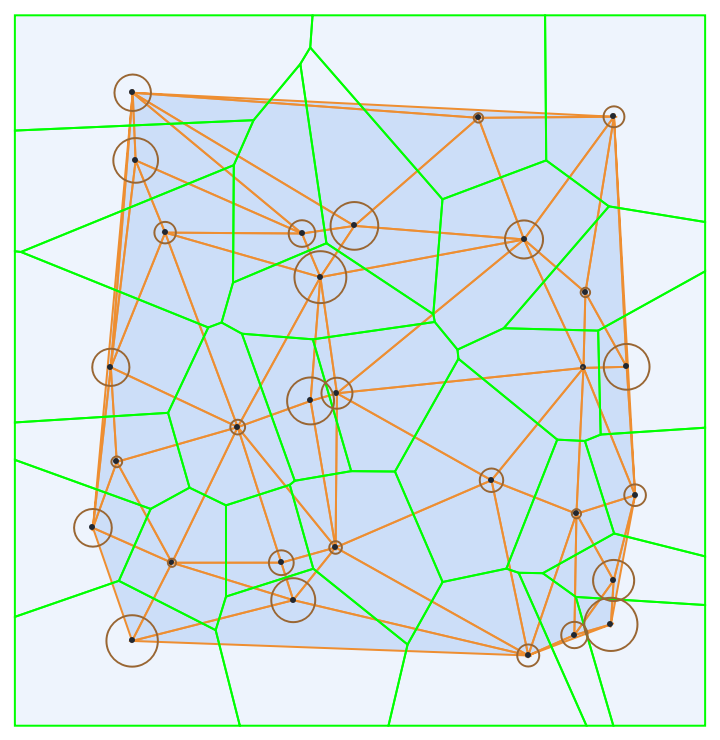Function Repository Resource:

PowerTriangulation

Generate the power triangulation of a set of circles

Contributed by: Jan Mangaldan
 ResourceFunction["PowerTriangulation"][{c1,c2,…}] gives a MeshRegion representing the power triangulation of the circles c1,c2, ….

Details

The circles ci can be Circle or Disk objects.
Point objects are treated as circles with zero radius.
A power triangulation is also known as Laguerre triangulation or Laguerre–Delaunay triangulation; it generalizes the Delaunay triangulation.
A power triangulation can be considered as a weighted Delaunay triangulation of the circles' centers, with the radii of the circles as weights.
A power triangulation consists of triangles (in 2D) and n-dimensional simplices (in nD).
An nD power triangulation has simplex cells defined by n+1 circle centers, such that the circumsphere for the same n+1 points contains no other circle centers from the original circles ci.
ResourceFunction["PowerTriangulation"] takes the same options as MeshRegion.

Examples

Basic Examples (2)

Generate the power triangulation of a set of circles:

 In:=Out=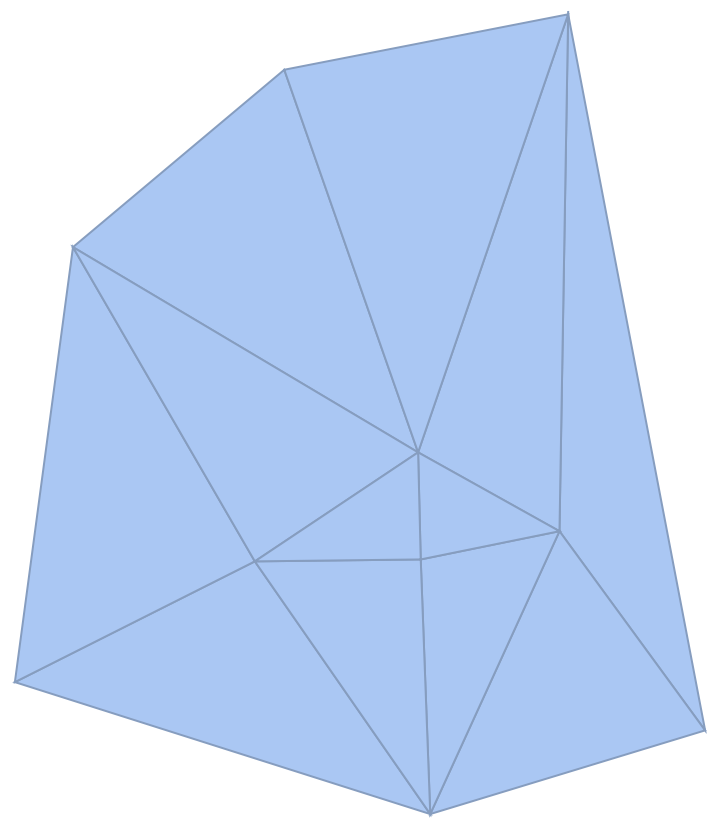Show the power triangulation with the generating circles:

 In:=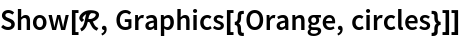Out=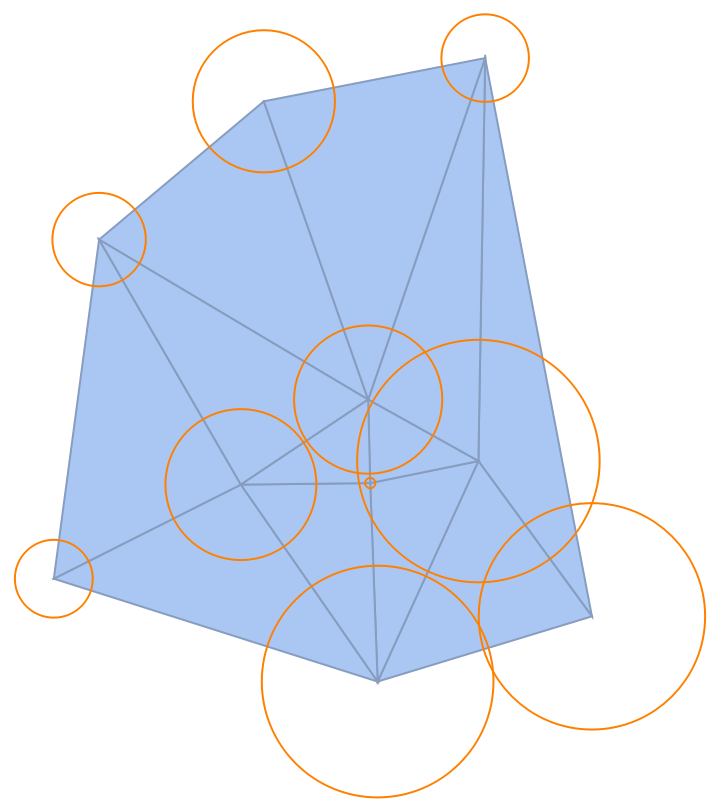Scope (2)

Create a power triangulation from a set of disks:

 In:=Out=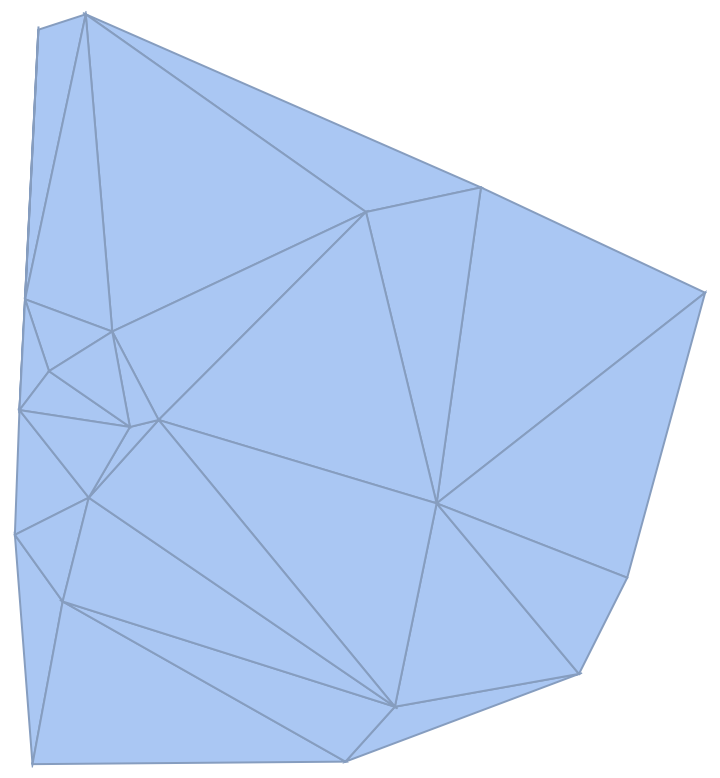Basic properties:

 In:=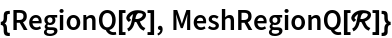Out=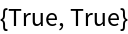Power triangulations are full dimensional:

 In:=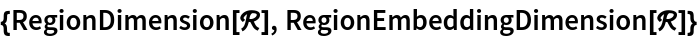Out=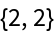Power triangulations are bounded:

 In:=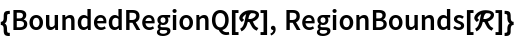Out=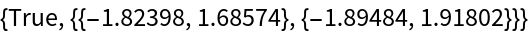Find its area and centroid:

 In:=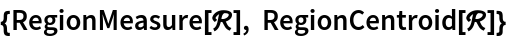Out=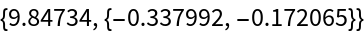Test for point membership or distance to the closest point in the region:

 In:=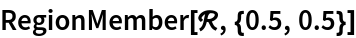Out=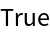In:=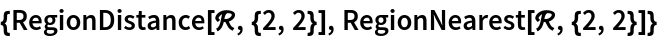Out=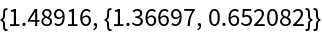Generate the power triangulation of a mixture of Circle and Disk objects:

 In:=Out=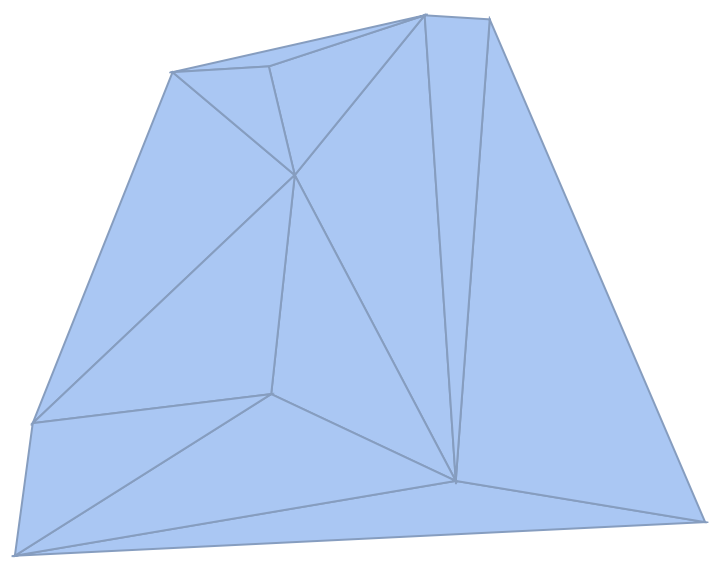Options (10)

MeshCellHighlight (2)

MeshCellHighlight allows you to specify highlighting for parts of a PowerTriangulation:

 In:=Out=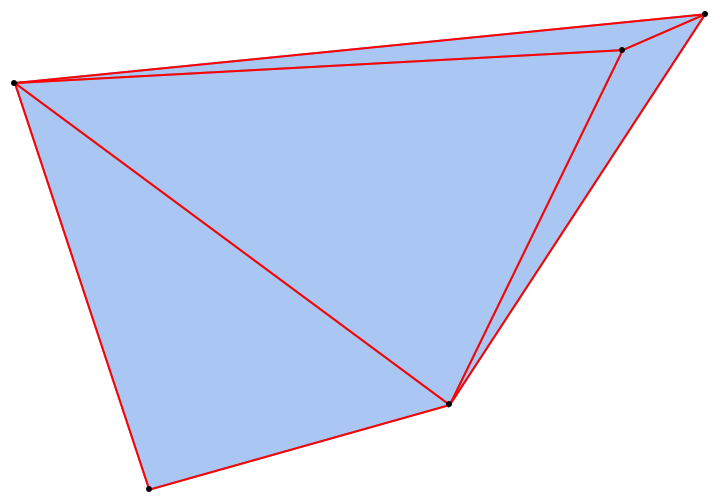Individual cells can be highlighted using their cell index:

 In:=Out=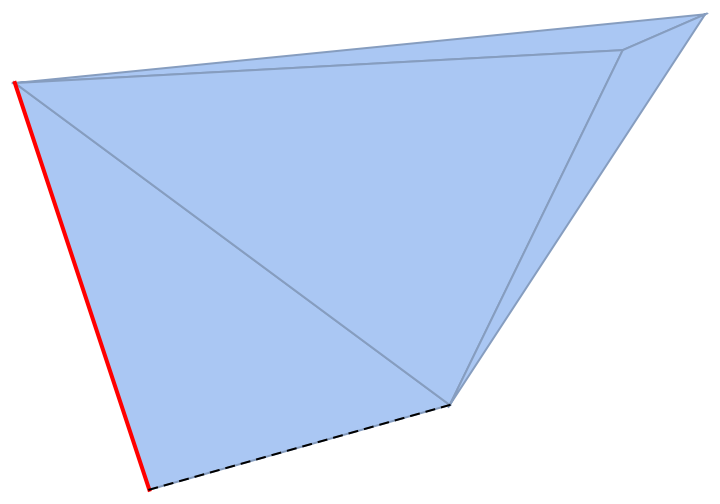Or by the cell itself:

 In:=Out=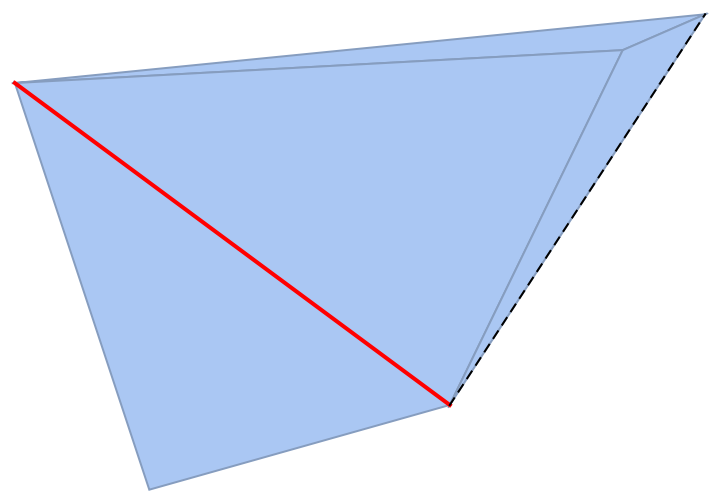MeshCellLabel (2)

MeshCellLabel can be used to label parts of a PowerTriangulation:

 In:=Out=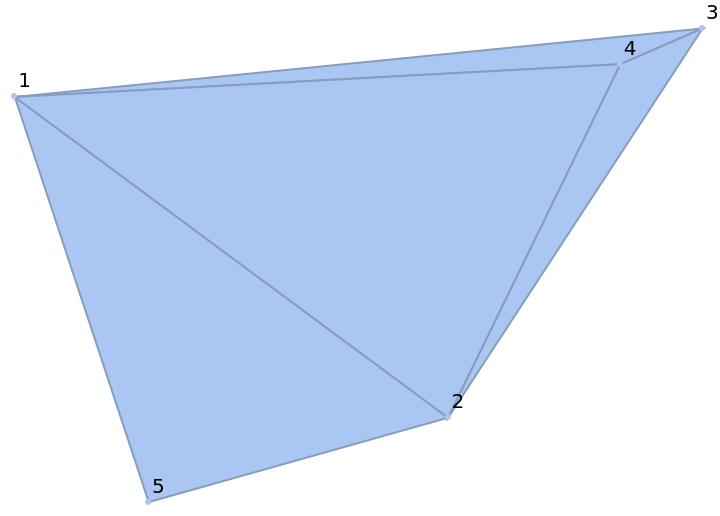Individual cells can be labeled using their cell index:

 In:=Out=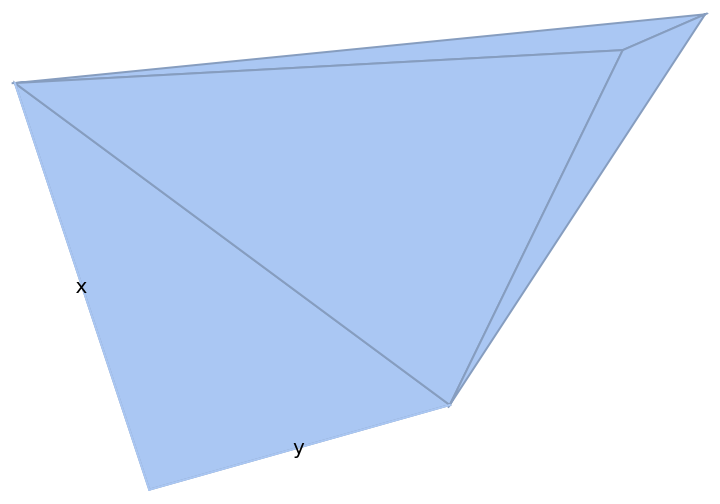Or by the cell itself:

 In:=Out=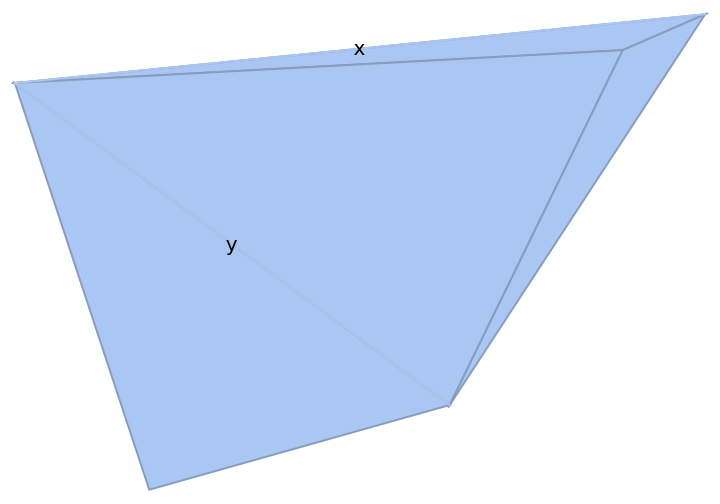MeshCellMarker (2)

MeshCellMarker can be used to assign values to parts of a PowerTriangulation:

 In:=Out=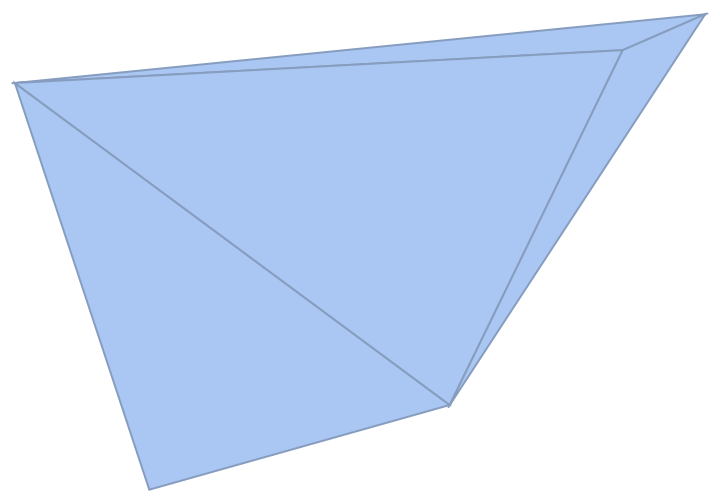Use MeshCellLabel to show the markers:

 In:=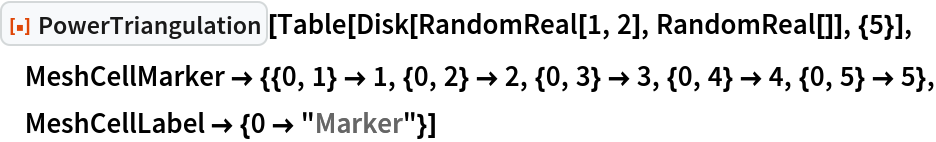Out=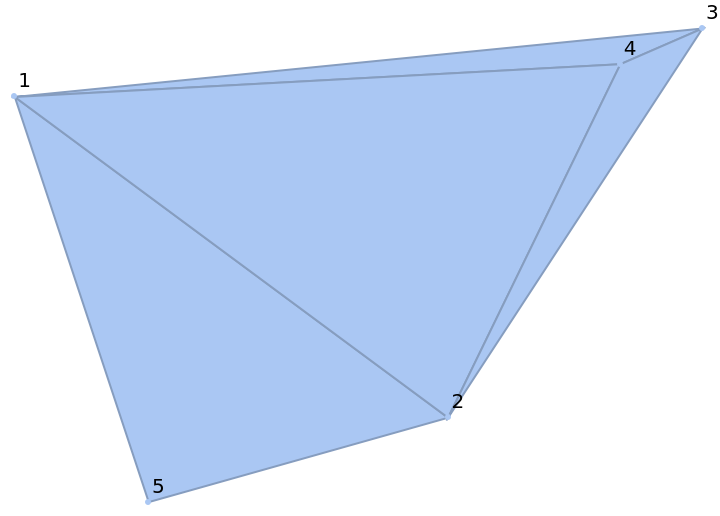MeshCellStyle (2)

MeshCellStyle allows you to specify styling for parts of a PowerTriangulation:

 In:=Out=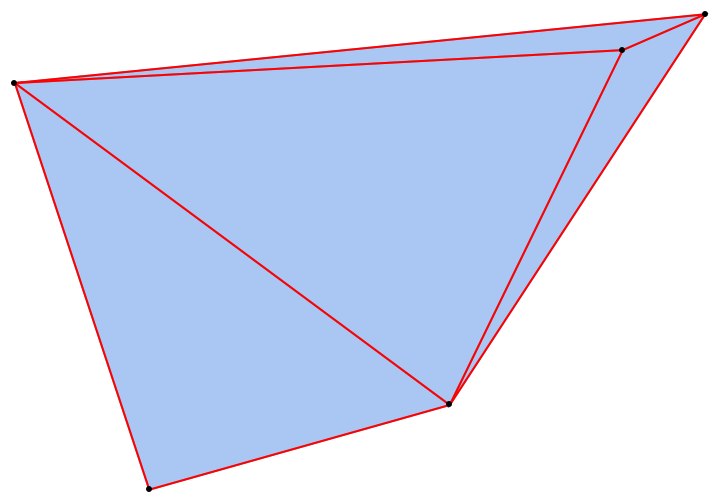Individual cells can be highlighted using their cell index:

 In:=Out=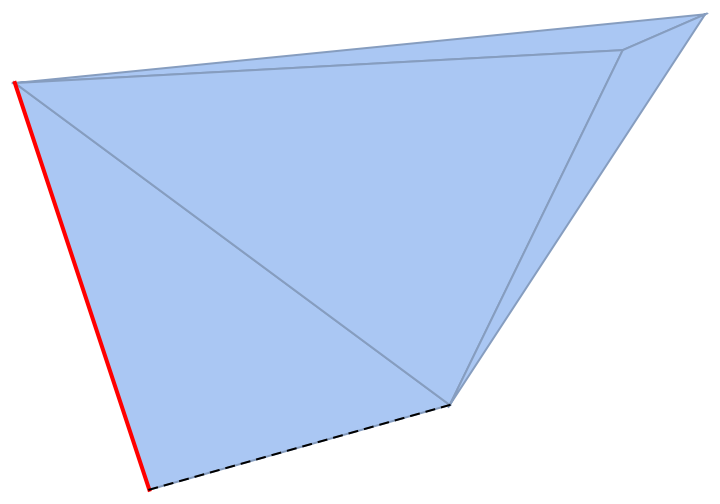Or by the cell itself:

 In:=Out=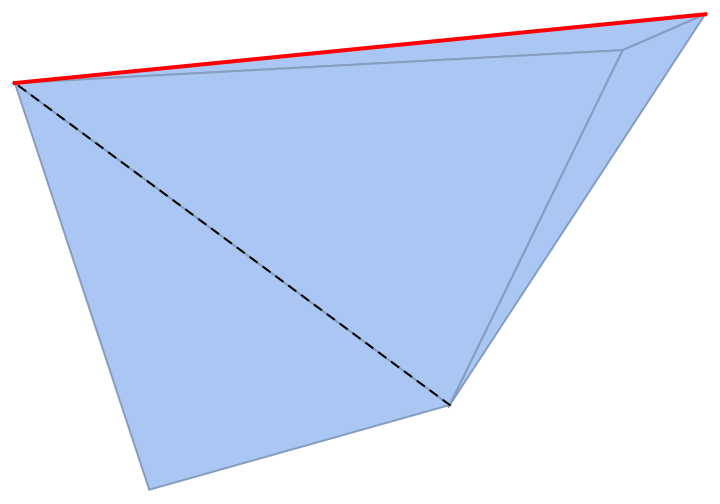PlotTheme (2)

Use a theme with grid lines and a legend:

 In:=Out=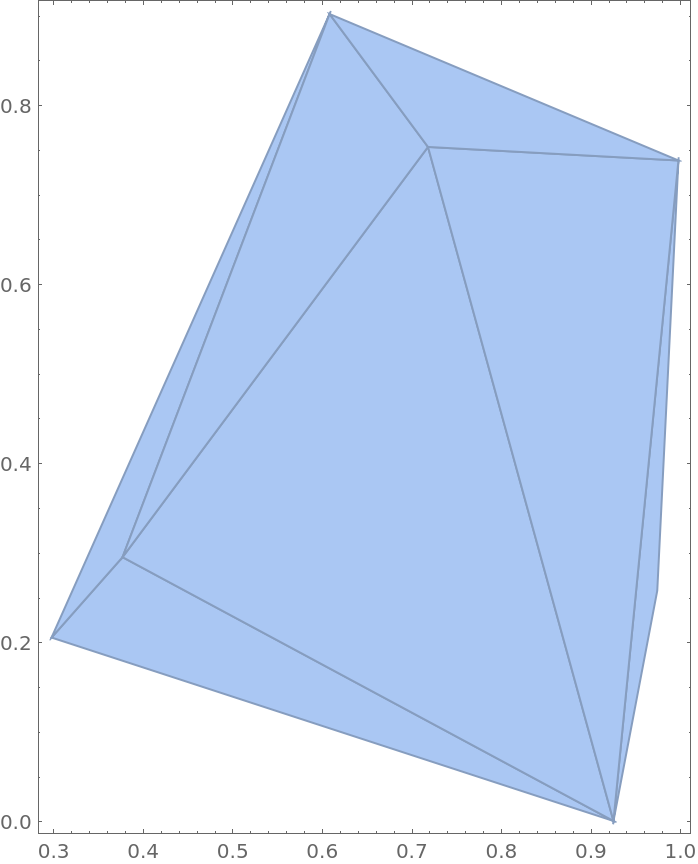Use a theme to draw a wireframe:

 In:=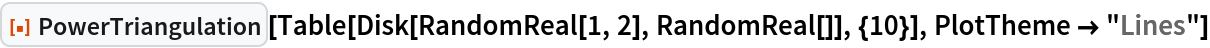Out=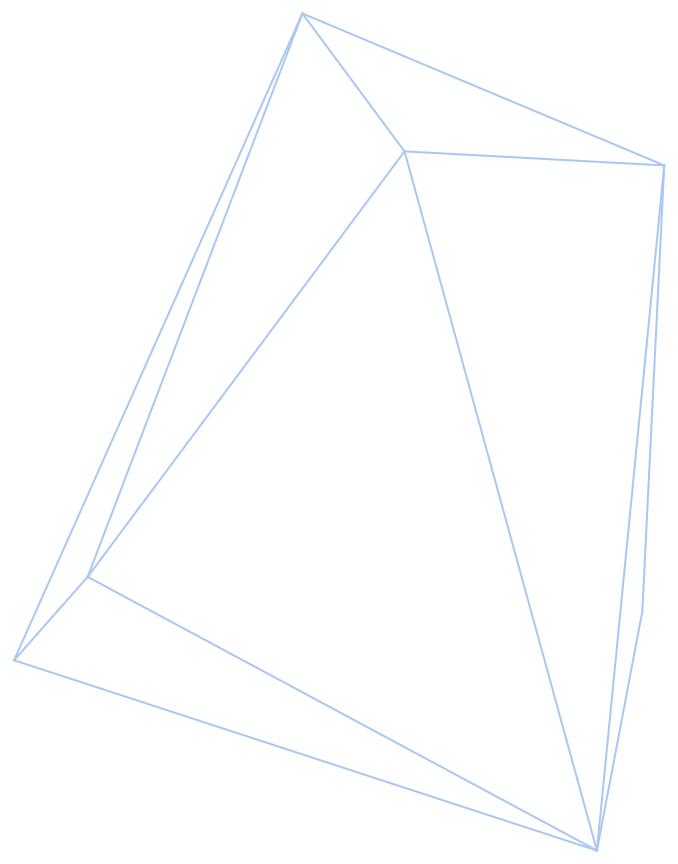Properties and Relations (4)

The output of PowerTriangulation is always a full-dimensional MeshRegion:

 In:=Out=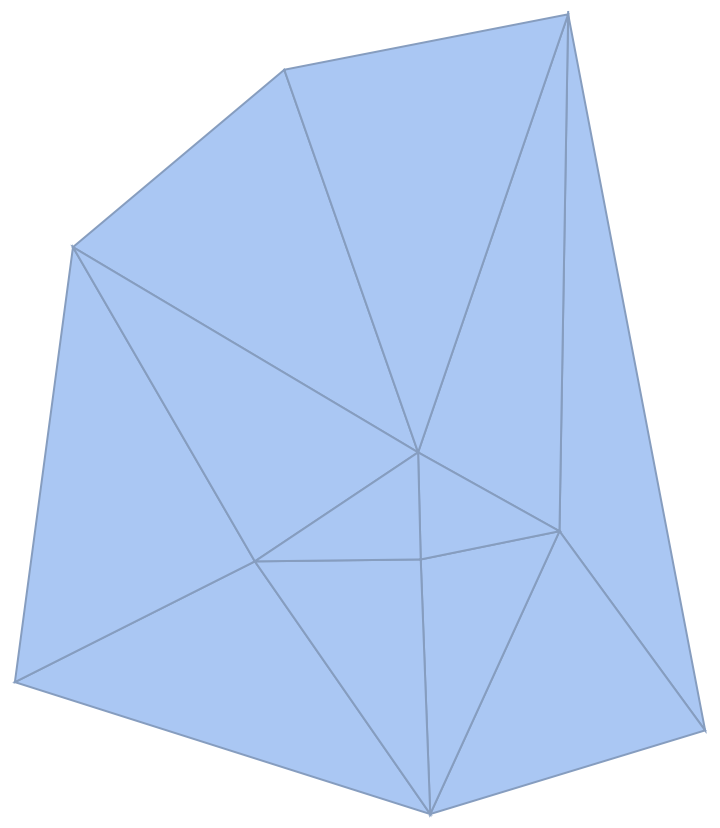In:=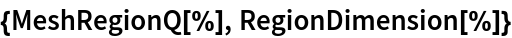Out=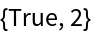The circumcircle for each triangle in a power triangulation contains no other circles' centers:

 In:=Out=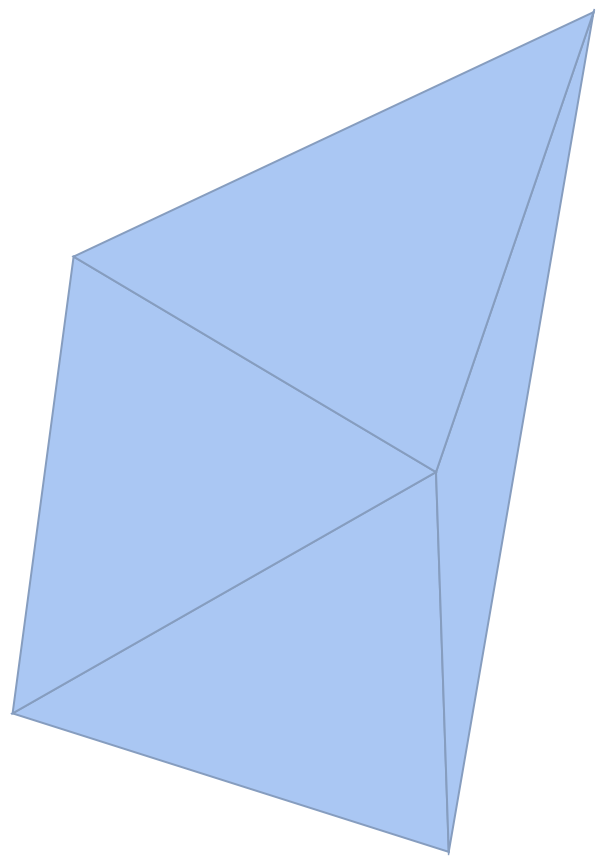Find circumcircles for all triangles:

 In:=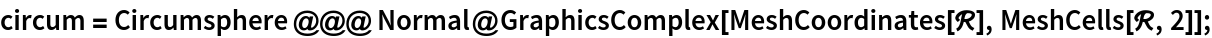Plot the circumcircles as disks:

 In:=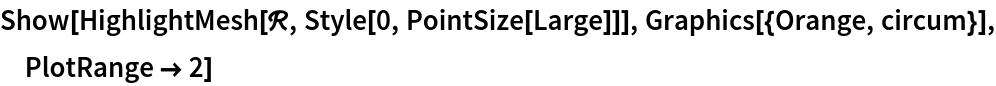Out=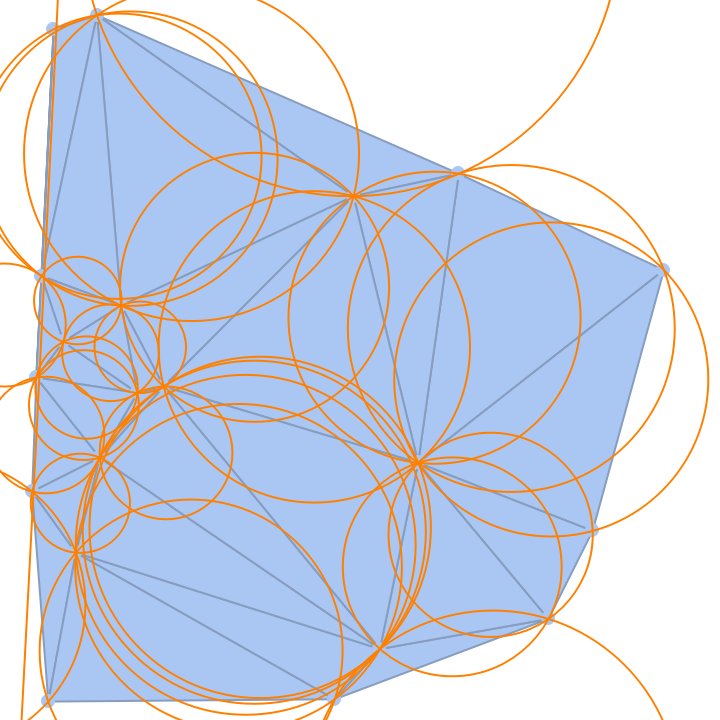The power triangulation of a set of circles all having the same radii is equivalent to the DelaunayMesh of the circles' centers:

 In:=Out=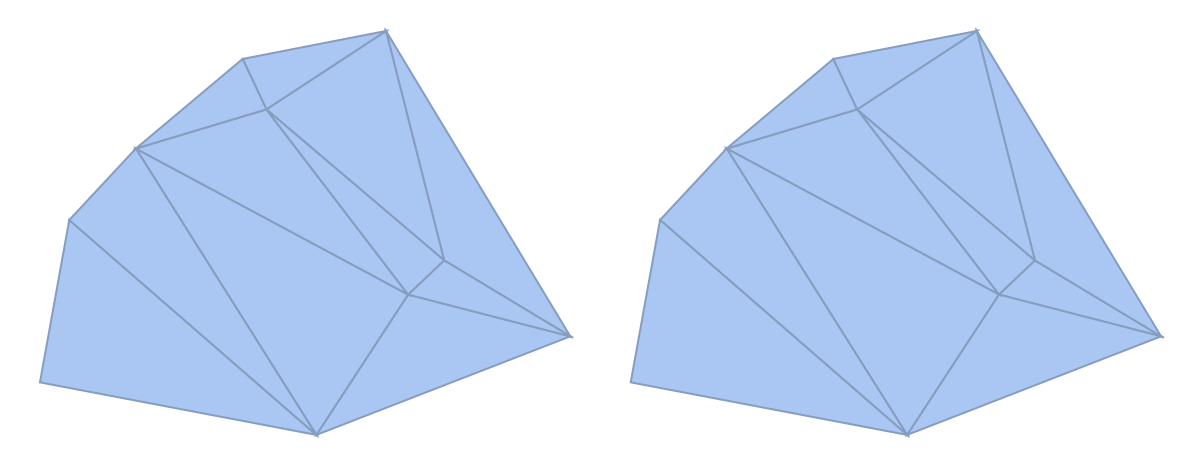The resource function PowerDiagram is the dual of PowerTriangulation:

 In:=Out=